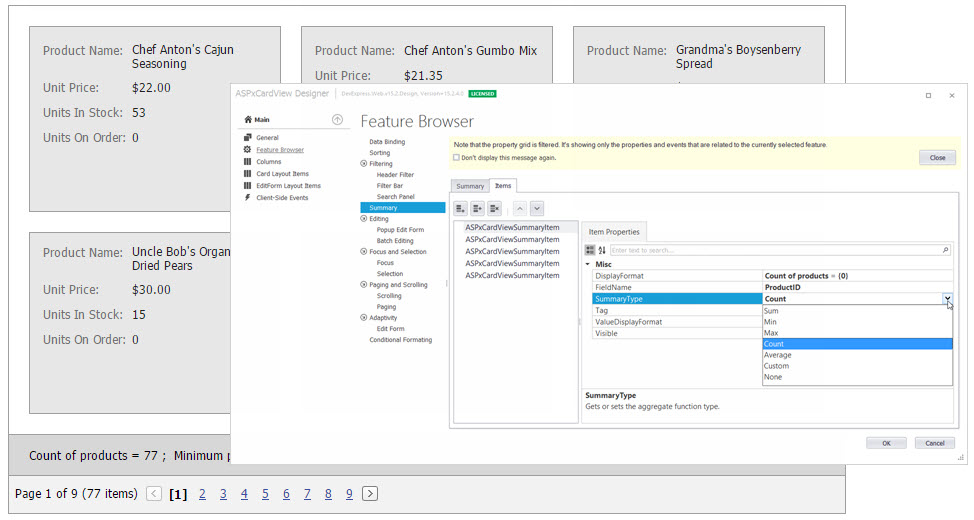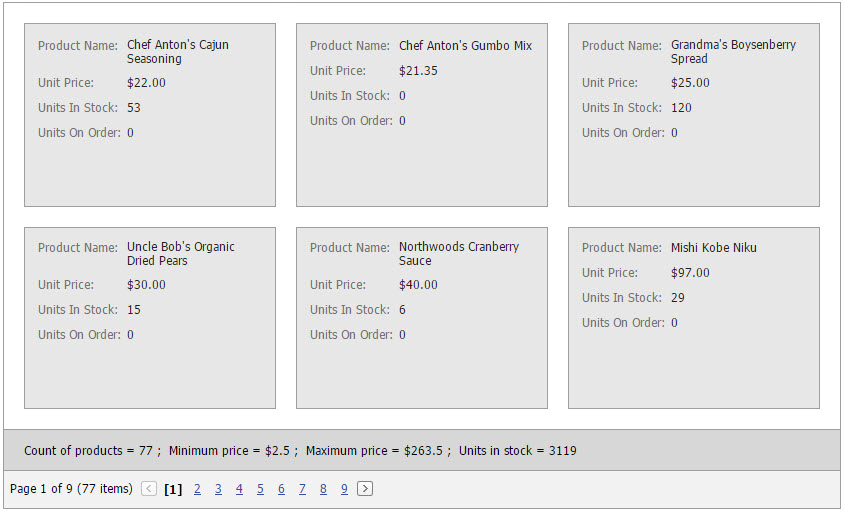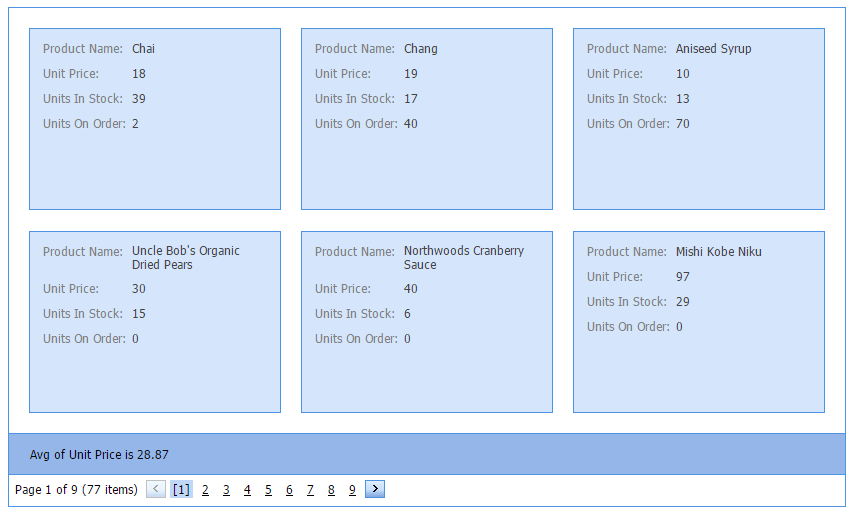# Total Summary

The total summary feature shows the value of an aggregate function calculated across all cards in ASPxCardView. Total summaries are displayed in the summary panel if the ASPxCardViewSettings.ShowSummaryPanel option is enabled.Total summaries are represented by the ASPxCardViewSummaryItem objects. ASPxCardView stores its total summaries within the ASPxCardView.TotalSummary collection. To manage this collection at design time, you can use the Edit Form as shown above.

To obtain a total summary value, use the ASPxCardView.GetTotalSummaryValue method.

## Example: Creating Total Summaries in Markup

The code sample below demonstrates how to specify total summaries.

The image below shows the result.``````<dx:ASPxCardView ID="ASPxCardView1"  runat="server" AutoGenerateColumns="False" DataSourceID="SqlDataSource1" KeyFieldName="ProductID">
<Settings ShowSummaryPanel="True" />
<Columns>
...
</Columns>
<TotalSummary>
<dx:ASPxCardViewSummaryItem FieldName="ProductID" SummaryType="Count" DisplayFormat="Count of products = {0} " />
<dx:ASPxCardViewSummaryItem FieldName="UnitPrice" SummaryType="Min" DisplayFormat="Minimum price = \${0} " />
<dx:ASPxCardViewSummaryItem FieldName="UnitPrice" SummaryType="Max" DisplayFormat="Maximum price = \${0} " />
<dx:ASPxCardViewSummaryItem FieldName="UnitsInStock" SummaryType="Sum" DisplayFormat="Units in stock = {0} " />
</TotalSummary>
</dx:ASPxCardView>
``````

## Example: Creating Total Summaries in Code

The following example calculates the average price and displays it in the ASPxCardView’s summary panel:``````ASPxCardViewSummaryItem totalSummary = new ASPxCardViewSummaryItem();
totalSummary.FieldName = "UnitPrice";
totalSummary.SummaryType = DevExpress.Data.SummaryItemType.Average;